Function Repository Resource:

# MatrixPolynomial

Evaluate a matrix polynomial

Contributed by: Jan Mangaldan
 ResourceFunction["MatrixPolynomial"][p,m] gives the matrix generated by the polynomial function p at the matrix argument m. ResourceFunction["MatrixPolynomial"][p,m,v] gives the matrix generated by the polynomial function p at the matrix argument m, applied to the vector v.

## Details

ResourceFunction["MatrixPolynomial"][p,m] effectively evaluates the polynomial function p with ordinary powers replaced by matrix powers.
ResourceFunction["MatrixPolynomial"][{c0,c1,,cn},m] with scalar ci takes the polynomial function to be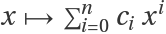.
ResourceFunction["MatrixPolynomial"] works only on square matrices.
ResourceFunction["MatrixPolynomial"] can be used on SparseArray objects.

## Examples

### Basic Examples (1)

Compute a matrix polynomial, specifying the polynomial as a pure function:

 In:=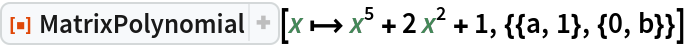Out=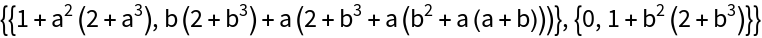### Scope (5)

Evaluate a matrix polynomial with a machine-precision matrix:

 In:=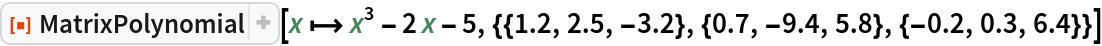Out=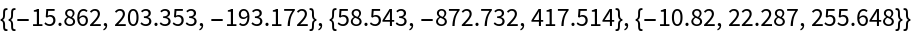The polynomial can be specified as a list of coefficients:

 In:=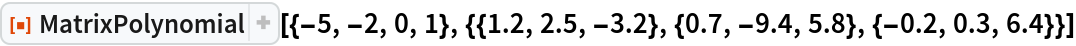Out=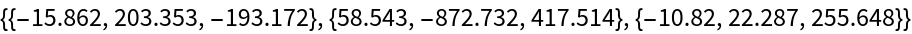Evaluate a matrix polynomial with a complex matrix:

 In:=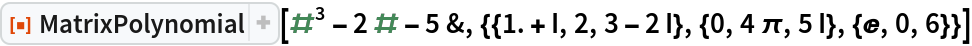Out=Evaluate a matrix polynomial with an arbitrary-precision matrix:

 In:=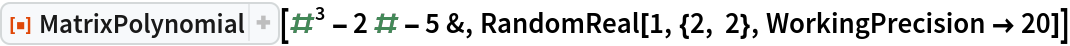Out=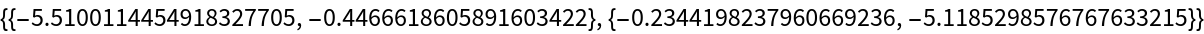Evaluating a matrix polynomial with a large machine-precision matrix is efficient:

 In:=Out=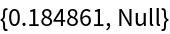Directly applying the matrix polynomial to a single vector is even more efficient:

 In:=Out=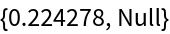Compute a matrix polynomial applied to a vector for a sparse matrix:

 In:=Out=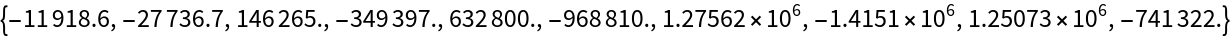### Applications (2)

Evaluate a matrix rational function:

 In:=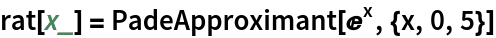Out=In:=Out=Compare with the result of MatrixFunction:

 In:=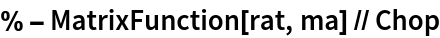Out=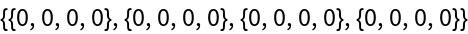### Properties and Relations (2)

MatrixPolynomial is more efficient than MatrixFunction:

 In:=Out=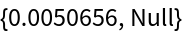In:=Out=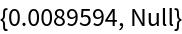If m is diagonalizable with m=v.d.v-1 and the eigenvectors are well conditioned, then p(m)=v.p(d).v-1:

 In:=Out=In:=Out=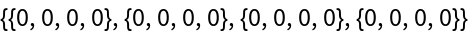### Neat Examples (1)

Demonstrate the Cayley–Hamilton theorem:

 In:=Out=In:=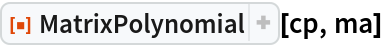Out=## Version History

• 1.0.0 – 08 March 2021